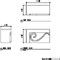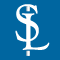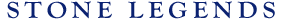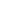HOMEPRODUCTSIDEA CENTERSERVICESTECHNICALABOUT USHello Guest Login  |  Become Member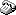800-398-1199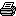214-398-1293MapEmail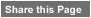Click to Share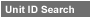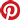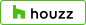Select which variables you would like to search by.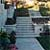Type DescriptionPrimary ViewPrimary ModifierUnit NameView Definitions

View Definitions

View DefinitionsX-Dim Y-Dim Z-Dim XX-Dim YY-Dim ZZ-Dim R-Dim D-Dim P-Dim
 Click column heading to re-sort results list.Product Name Primary View Modifier Pri Dimx  y  z Alt Dimxx  yy  zz Alt2 Dimr  d  p E\$timated Thumbnail Bolson Tread 2 Steps Straight X = 2-1/8" Y = 12"Z = 13-1/8" XX = 1-1/2" YY = n/aZZ = 12" R = n/a D = n/aP = n/a \$ 14.25Bolson Tread 3 Steps X = 4-1/2" Y = 12"Z = 13-1/2" XX = 3" YY = n/aZZ = 11" R = n/a D = n/aP = n/a \$ 17.94Bolson w/cove riser Steps X = 10" Y = 12"Z = 13" XX = n/a YY = n/aZZ = n/a R = n/a D = n/aP = n/a \$ 20.00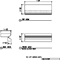Bullnose 2" Steps Straight X = 2" Y = 36"Z = 14" XX = n/a YY = n/aZZ = n/a R = n/a D = n/aP = n/a \$ 8.40Bullnose 2" Tread Steps Treads Riser X = 2" Y = 12"Z = 14" XX = 1-3/4" YY = n/aZZ = 13-1/8" R = n/a D = n/aP = n/a \$ 15.40Bullnose 2.25" Tread Steps Straight X = 2-1/4" Y = 12"Z = 14" XX = n/a YY = n/aZZ = n/a R = n/a D = n/aP = n/a \$ 15.40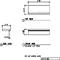Bullnose 2.5" Tread Steps X = 2-1/2" Y = 12"Z = 14" XX = n/a YY = n/aZZ = n/a R = n/a D = n/aP = n/a \$ 17.97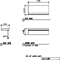Bullnose Tread Steps Straight X = 2" Y = 36"Z = 15" XX = n/a YY = n/aZZ = 14" R = n/a D = n/aP = n/a \$ 100.00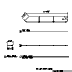Bullnose Tread Angled Steps X = 8-1/2" Y = 12"Z = 14" XX = 2" YY = n/aZZ = 13" R = n/a D = n/aP = n/a \$ 15.40Bullnose With Radius 1 Steps X = 9-15/16" Y = 72-3/8"Z = 11-5/8" XX = 2-1/2" YY = n/aZZ = n/a R = 11" D = n/aP = n/a \$ 413.21Bullnose With Radius 2 Steps X = 2-1/2" Y = 12"Z = 35" XX = 2" YY = n/aZZ = n/a R = 11" D = n/aP = n/a \$ 24.36Bullnose With Radius 3 Steps X = 9-3/8" Y = 12"Z = 12" XX = n/a YY = n/aZZ = n/a R = 6" D = n/aP = n/a \$ 15.73Recurve1 Steps Straight Bracket X = 5" Y = 7-3/4"Z = 2" XX = n/a YY = n/aZZ = 1-1/4" R = n/a D = n/aP = n/a \$ 12.29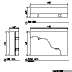Recurve2 Steps Straight Bracket X = 5" Y = 9-3/4"Z = 2" XX = n/a YY = n/aZZ = n/a R = n/a D = n/aP = n/a \$ 14.29Revel1 Steps Straight Bracket X = 6-3/8" Y = 10-5/8"Z = 4-1/2" XX = n/a YY = n/aZZ = n/a R = n/a D = n/aP = n/a \$ 37.50